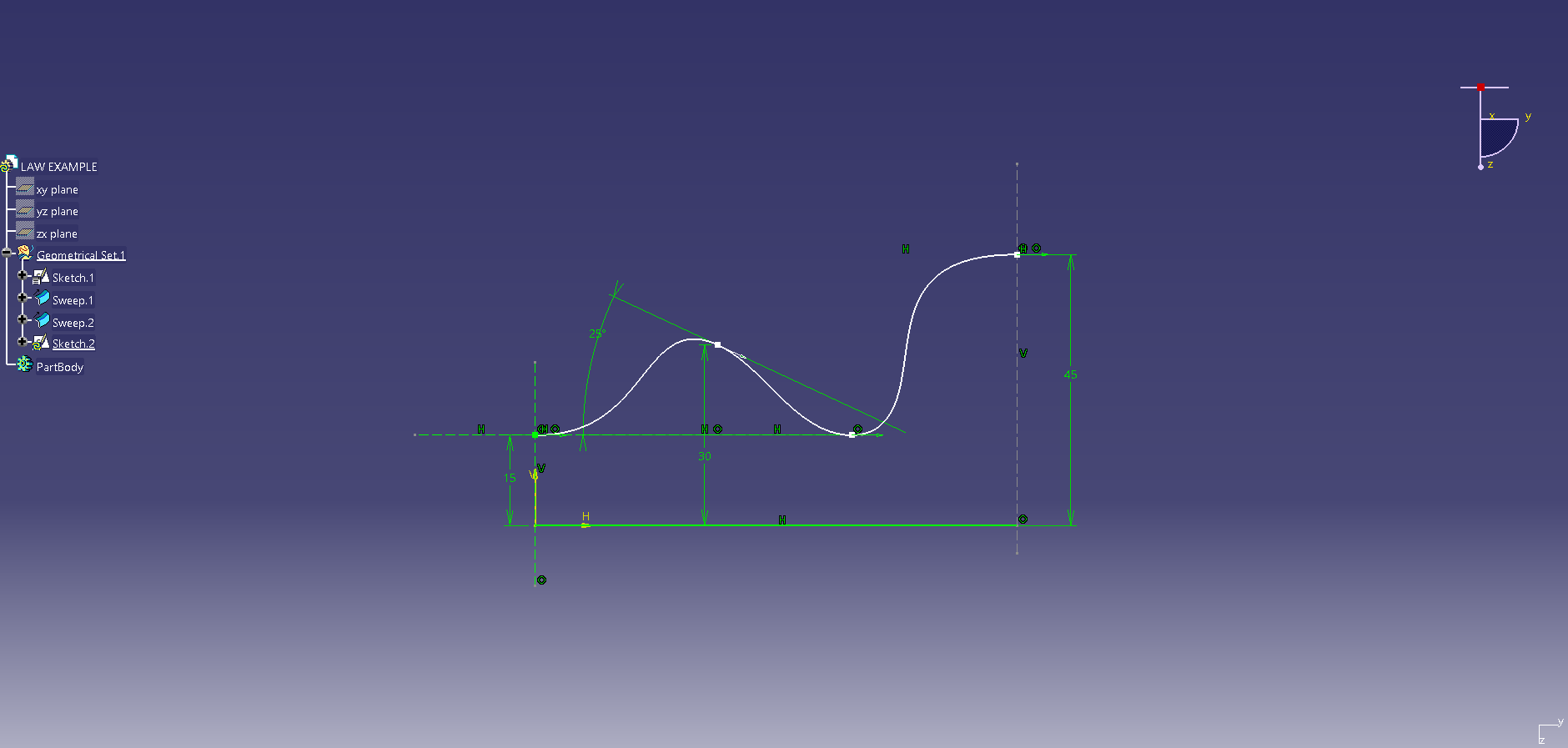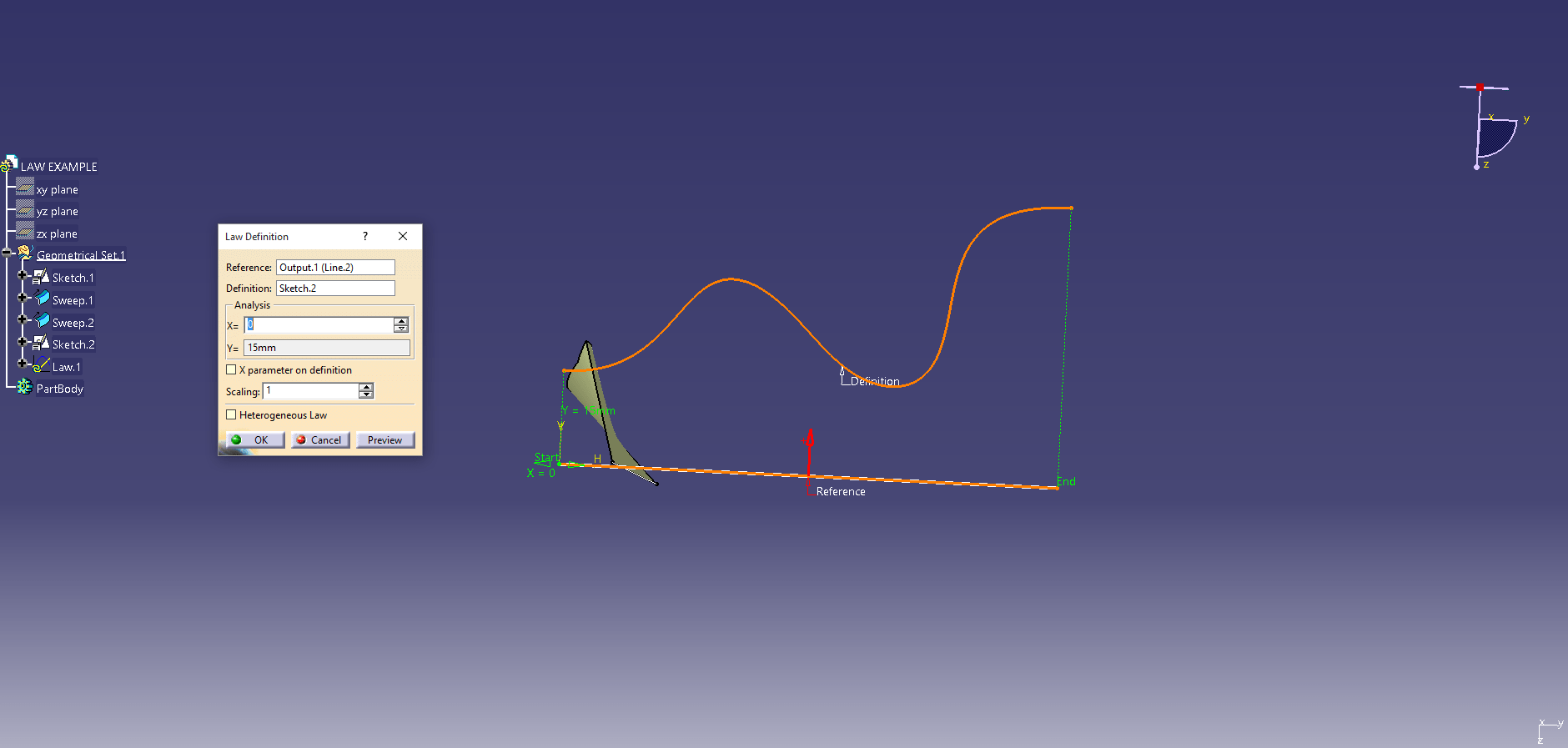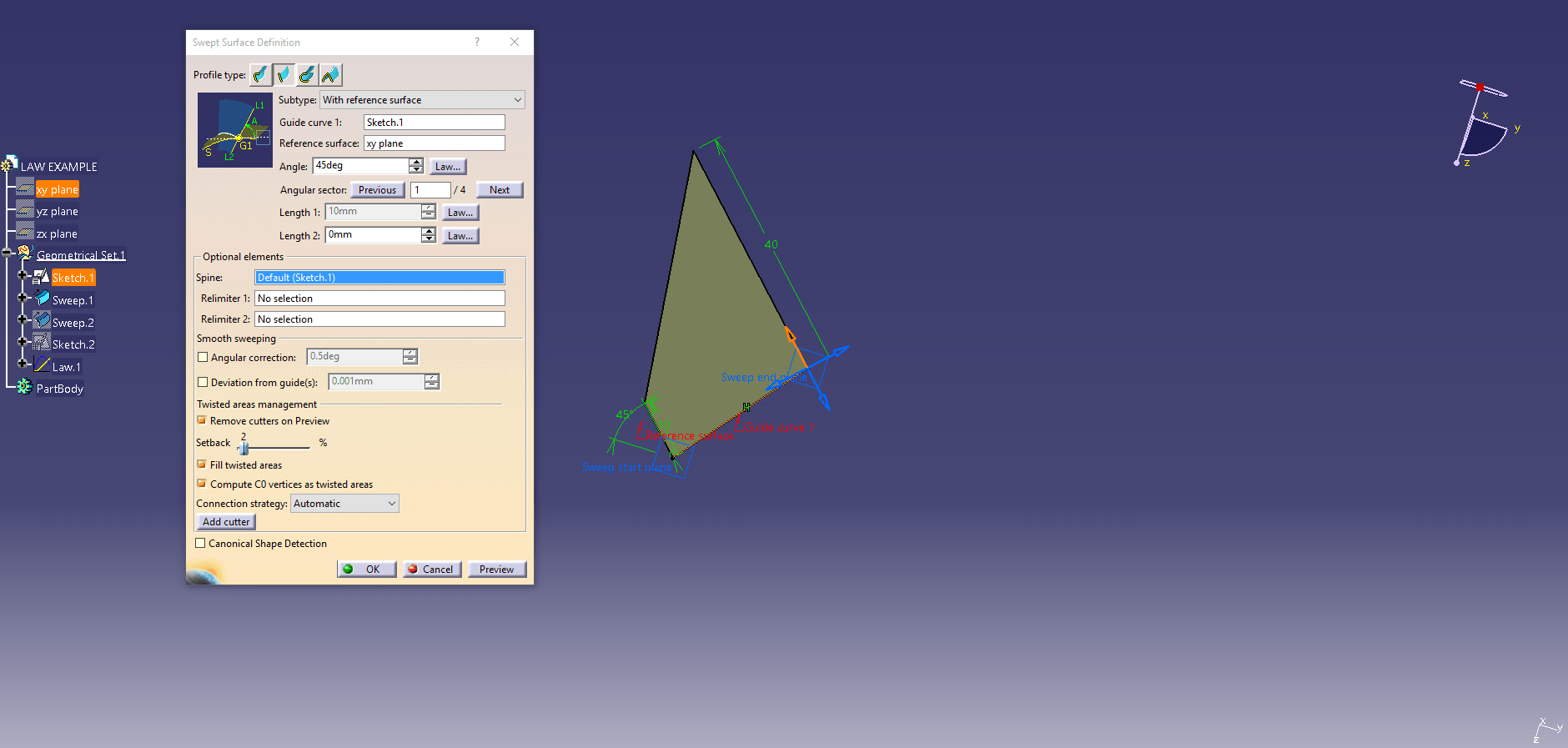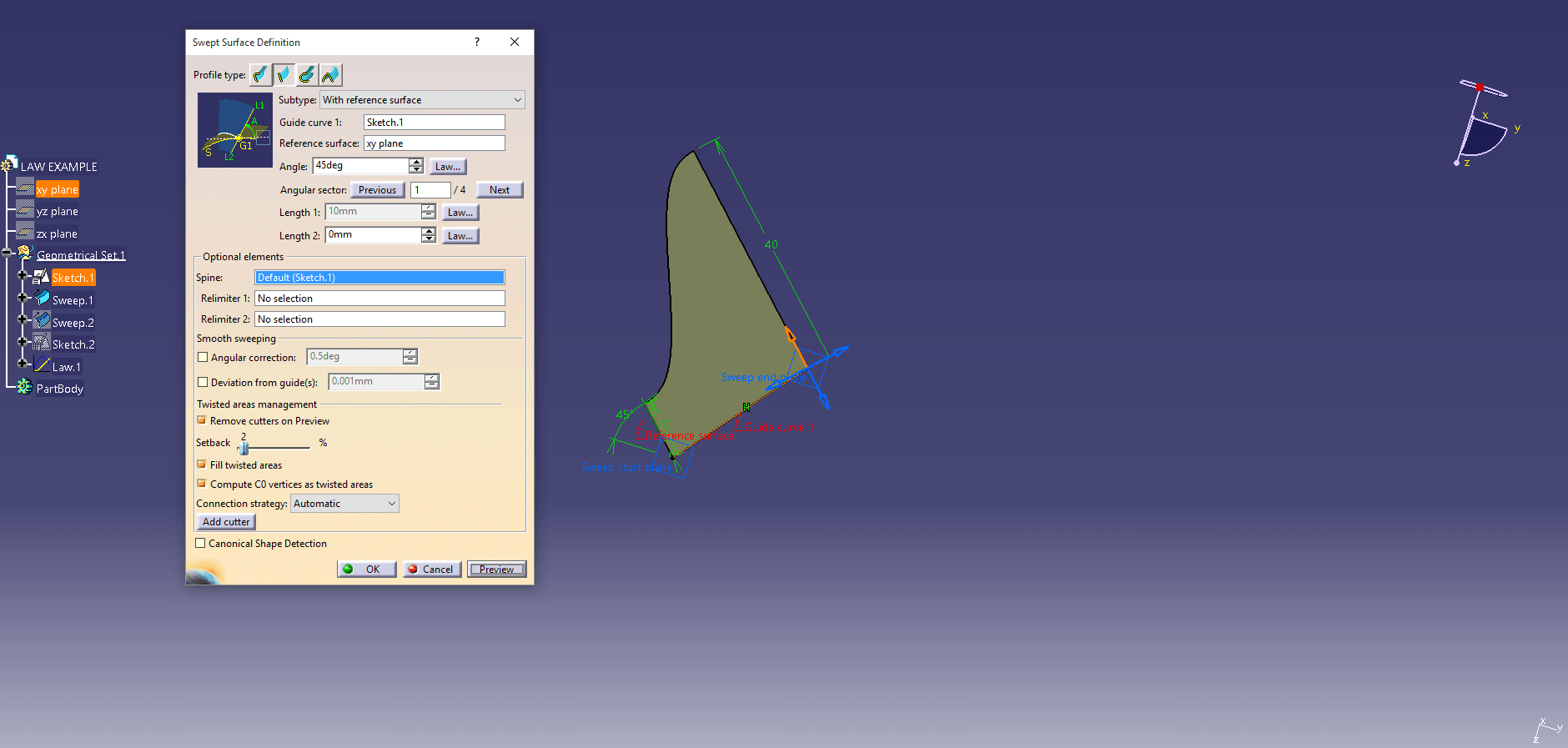## Laying down the Law! – Using Laws To Control Your Features

Laws are very useful when it comes to wanting to control something that has a known variance in it.  For example, if I were a designer and needed a linear surface that begins at one angle at one end of the guide curve and ends with a different angle. Without the ability to do this, you would have to create two surfaces and do some sort of transition surface in between them. In this video below, I will run a linear surface using a simple law on the angle value to take it from 15 degrees and one end to 45 degrees at the other end.

In this case I used a linear style law, and as you see, when I looked at the surface from a plan view (from above) the angle direction was linear from the 15 deg to the 45 deg.  Below, I will show what would have happened if I had done it as an “S Type” law by modifying the law.

In the image below you can see them if they are overlayed over each other. The surface highlighted is the S Type law and as you can see it definitely has an “S” shape for the transition in between the 2 knows angles.Both Law Types

You’re probably thinking, “What if I wanted a specific angle somewhere in the middle of the transition?” This gets a little trickier. In that case you would use an Advanced Law.

In order to used the advanced type law, you have to first develop it.  The easiest way I have found to do this is with a sketch.  In the example below, I am showing what the sketch would look like for the original linear law.Linear Law Sketch

The bottom green line would be considered the reference line or X=0 and Y=15. Eventually Y becomes 45.  These driving numbers would be the angle definition.

The “S Type” Law Sketch would look like the sketch below, where there would be a linear line at the 15 and a linear line at the 45 and a connect curve in between them.S Type Law Sketch

To develop a law that has various numbers to meet, you would simply follow the same method as S Type but use a spline curve to represent where you want the transition.  In this example below, I will add in a transition that goes from 15 to 30 and down to 15 and finish at 45.To build the law to attach to the sketch I recommend making one of the sketch lines an output feature so it can be picked separately in the Law command.Once the item is in the tree, you can use it anywhere you need a law that incorporates the variances of those values. Let’s add it to the linear surface created earlier and adjust the values as shown in the video below.

The last thing to show here is the law being used to control length instead of angle. Maybe we would want to have a constant angle value and and variance for the length.Length Controlled Linear LawLength Controlled S Type LawLength Controlled Advanced Law Created Earlier

As you can see the possibilities are endless.  You can control the values any way you want and they work the same anywhere in the software that you see the Law… button in a dialog.

Bottom line, if you need to design smarter and take control of your designs, Tata Technologies will help you lay down the law!